﻿ Letter Grid — Keep Talking and Nobody Explodes Module## On the Subject of Letter Grids

Guess you should've taken a foreign language in high school, huh?

• The module has one of every letter from A-Z excluding J in a 5×5 grid. However, each letter will be encrypted differently.
• The grid will contain one or more (albeit very rarely) words from a word list below.
• Select the row or column that has a word from the word list, spelled either forwards or backwards.
 Actor Brawl Curve Delta Exish Fired Grabs Hotel Inert Knife Lemon Might Nymph Ovals Plums Quoth Raids Squab Toxic Ulzie Veils Waved Xrays Yanks Zoned
• The following encryptions will be present on the module. Letters will be listed from A-Z in a 5×5 grid.
 Animals A B C D E F G H I K L   M N O P Q R S T U V W X Y Z Binary * 00001 | 00010 | 00011 | 00100 | 00101 00110 | 00111 | 01000 | 01001 | 01011 01100 | 01101 | 01110 | 01111 | 10000 10001 | 10010 | 10011 | 10100 | 10101 10110 | 10111 | 11000 | 11001 | 11010
 Bioblanner A B C D E F G H I K L M N O P Q R S T U V W X Y Z Boozleglyphs A B C D E F G H I K L M N O P Q R S T U V W X Y Z Braille A B C D E F G H I K L M N O P Q R S T U V W X Y Z CMP(? and ! are not used) a p' C t' e f k' h i k r' m n o p ? r s t u f' w ! y s'
 Cube Symbols A B C D E F G H I K L M N O P Q R S T U V W X Y Z Dancing Men A B C D E F G H I K L M N O P Q R S T U V W X Y Z Decoborders A B C D E F G H I K L M N O P Q R S T U V W X Y Z Elder Futhark(K and W will never show up.) a b c d e f g h i k l m n o p q r s t u v w x y z
 English A B C D E F G H I K L M N O P Q R S T U V W X Y Z Hieroglyphics(H is representedby the colons listed,F and X will neverbe encrypted.) A B C D E F G H I K L M N O P Q R S T U V W X Y Z Lombax A B C D E F G H I K L M N O P Q R S T U V W X Y Z Maritime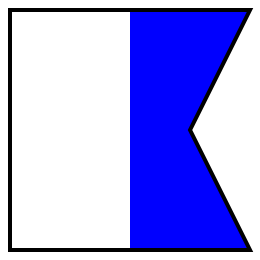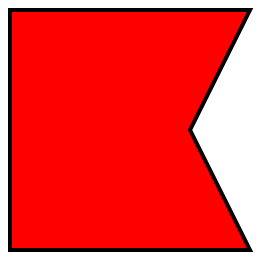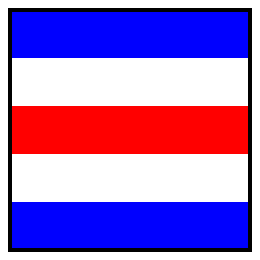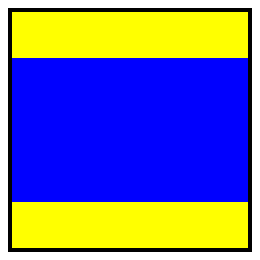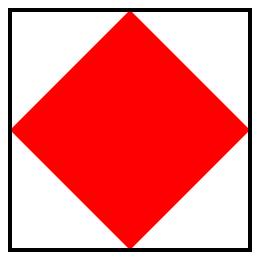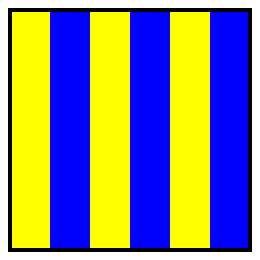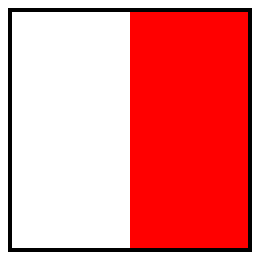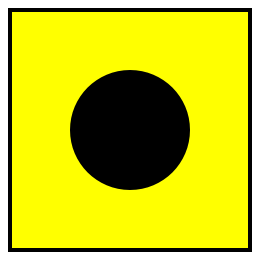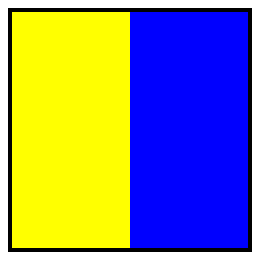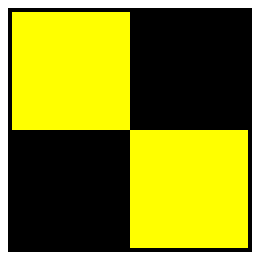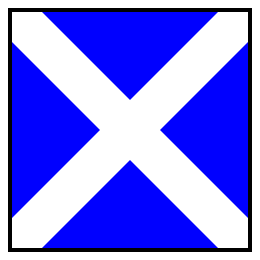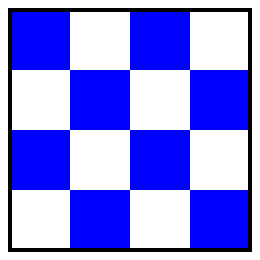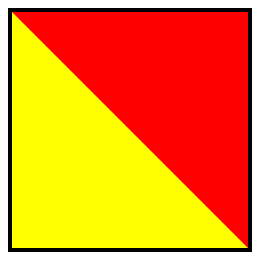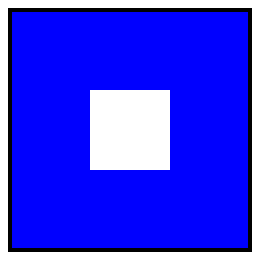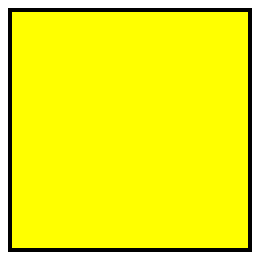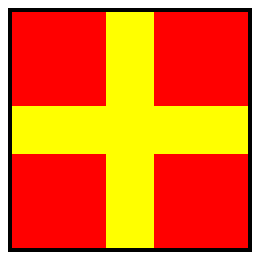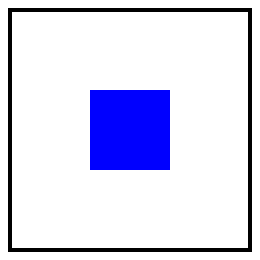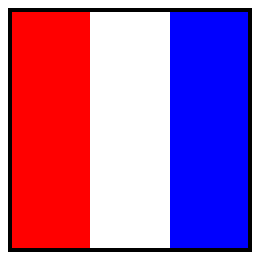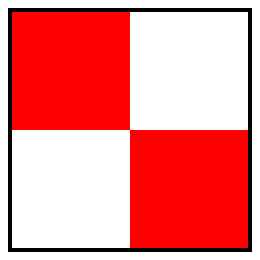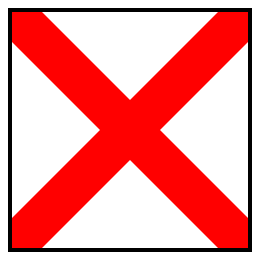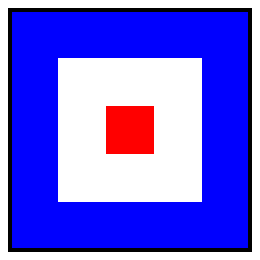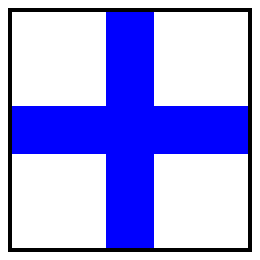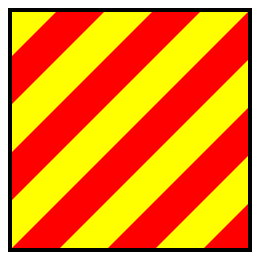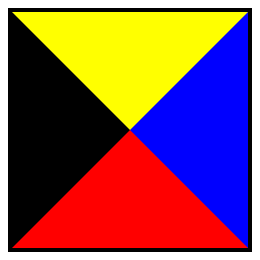Moon Type A B C D E F G H I K L M N O P Q R S T U V W X Y Z More Code ** .-.,. | = | =,, | =,- | .,.-= -.- | . | ,.. | =--= | , =-,= | ,,. | --.= | ----- | - ==. | ,--= | -,== | =.,, | ===== =, | ,= | =. | -, | ,=- Morse Code *** .- | -... | -.-. | -.. | . ..-. | --. | .... | .. | -.- .-.. | -- | -. | --- | .--. --.- | .-. | ... | - | ..- ...- | .-- | -..- | -.-- | --.. Pigpen A C E G I K M O Q D F H J L N P R S U W Y T V X Z
 R'lyehian a b c d e f g h i k l m n o p q r s t u v w x y z Semaphore(N will not be used.) A B C D E F G H I K L M \ O P Q R S T U V W X Y Z Semaphore Telegraph A B C D E F G H I K L M N O P Q R S T U V W X Y Z Standard Galactic Alphabet A B C D E F G H I K L M N O P Q R S T U V W X Y Z
 Tap Code **** 11 12 13 14 15 21 22 23 24 66 31 32 33 34 35 41 42 43 44 45 51 52 53 54 55 Wingdings A B C D E F G H I K L M N O P Q R S T U V W X Y Z Zoni A B C D E F G H I K L M N O P Q R S T U V W X Y Z
• * A number will be on the square, representing the bit from left to right. A gray coloring represents a 1, and black represents a 0.
• ** The green square will be representing More Code.
• *** The red square will be representing Morse Code.
• **** The blue square will be representing Tap Code.